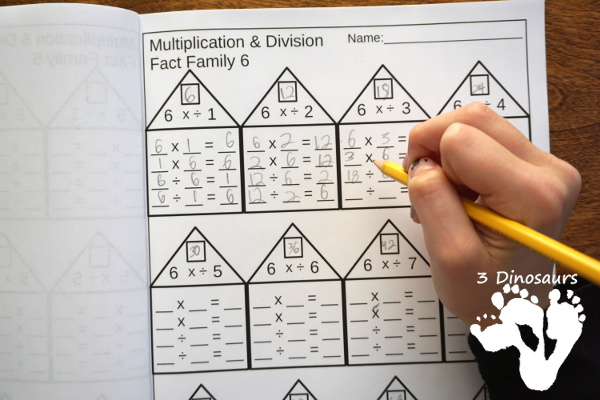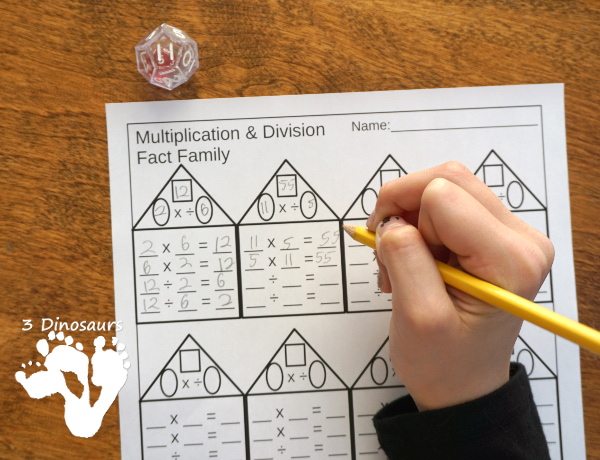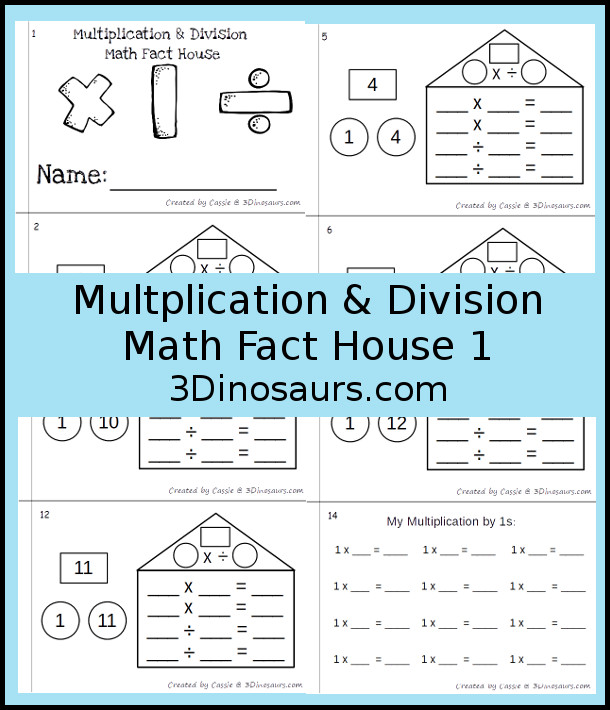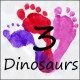Multiplication & Division Math Fact House Books

Math facts have to be one of the hard things for some kids to work on. We have used the math fact houses a lot. I wanted to work on that worked with those printables.

At the same time, I have two girls that love things that come in books. They love flipping through pages and learning something.

So, I put the math facts and books together into Multiplication & Division Math Fact House Books!There are two levels of the books: Beginner Math Fact Books and Review Math Fact Books. I did this before beginners become ones who need will soon need to review their math facts.

Beginner Multiplication & Division Math Fact Books

The beginner books have a single book for each number 1 through 12. It was a way to give kids a single set of numbers to work on.

Each of the pages shows three numbers that work together to fill out a math fact house.The last two pages of the book are the equations you can fill out for multiplication and division. It has space for kids to fill out all 12 equations that they can find in their book.One nice thing about the books is that once the books are finished, you have great review books kids can look through.

Review Multiplication & Division Math Fact Books

I know that last winter I was pulling my hair out for ideas for a review for winter break of multiplication. We did tons of math fact house activities but we had no real way to keep track of what she did or something that made it fun.

There are three sets of pages in this book set: blank, 1 number of the number bond filled out, and 2 numbers of the number bond filled out. I printed out one whole set of the pages and made a review book for my middle on her math review days.But all these pages can be just used as a single page for kids to work on as well. The blank page works great for a center. You can use it with the math fact houses to fill out what numbers you use.

I like to give my older girls a 12-sided dice in diceto fill out the blank page. It is easy and no-prep needed at all!What you will find in the Multiplication & Division Math Fact Books printables:

The set is a collection of two types of books: Beginner Multiplication & Division Math Fact Books and Review Multiplication & Division Math Fact Books. Both books give kids a chance to work on learning and reviewing their math fact for multiplication and division.What you will find in these printables:

• Math facts for Multiplication & Division from 1 to 12
• 12 Beginner Multiplication & Division Math Fact Books
• Single Print and Teacher Print Options for the Math Fact Books
• 15 pages for each begginner books
• 3 pages Types for the Review Multiplication & Division Math Fact Books
• Blank
• 1 number of the number bond filled out
• 2 numbers of the number bond filled out
• Option to use as a No-Prep Printable for the Review Multiplication & Division Math Fact Books
• 316 pages of printables
• Price: \$4.50

Multiplication & Division Math Fact House Books Sample

I have a sample of number 1 easy reader book for you to check out.

What you will find in these printables

• Multiplication & Division Math Fact Number 1
• 9 pages of printablesCassie – 3Dinosaurs.com